Function Repository Resource:

# FindAllPermutations

Find all permutations of lists with non-unique elements

Contributed by: Nikolay Murzin
 ResourceFunction["FindAllPermutations"][list] returns a list of permutations that produces a list from its sorted version. ResourceFunction["FindAllPermutations"][list1,list2] returns a list of permutations that permutes list1 into list2.

## Details

Permutations are returned as Cycles.

## Examples

### Basic Examples (2)

Find all permutations that produce a list with non-unique elements from its sorted version:

 In:=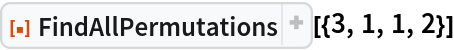Out=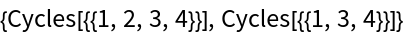Find all permutations that convert one list to another:

 In:=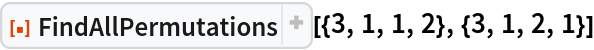Out=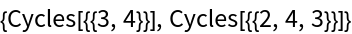### Scope (1)

If a list can't be permuted into a target list, an empty list is returned:

 In:=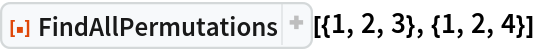Out=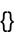### Properties and Relations (2)

FindPermutation only finds one permutation:

 In:=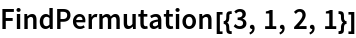Out=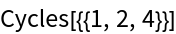FindAllPermutations finds all possible permutations:

 In:=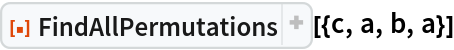Out=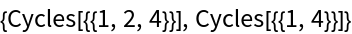## Version History

• 1.0.0 – 13 December 2021